### 文章目录

BA本质上是一个非线性优化算法，先来看看它的原型
min ⁡ x ∑ i ρ i ( ∣ ∣ f i ( x i 1 , x i 2 , . . . , x i k ) ∣ ∣ 2 ) \min_x \sum_i{\rho_i(||f_i(x_{i1}, x_{i2}, ..., x_{ik})||^2)}

f ( K i , R i , T i , P j ) = π ( K i [ R i    T i ] P j ) − p j i f(K_i, R_i, T_i, P_j)=\pi(K_i[R_i\ \ T_i]P_j) - p_j^i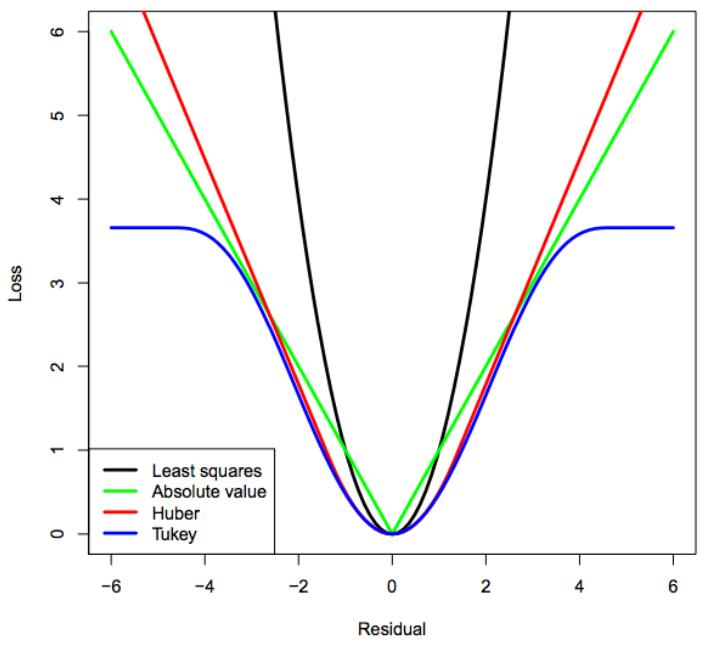# Ceres Solver

Ceres Solver专为求解此类问题进行了大量的优化，有很高的效率，尤其在大规模问题上，其优势更加明显。并且，Ceres内置了一些常用的函数，比如对坐标的旋转以及各类损失函数，使其在开发上也比较高效。在官网上可以找到它的编译方法和使用教程，Windows用户可以在此找到配置好的VS工程。

# 编写代码

struct ReprojectCost
{

cv::Point2d observation;

ReprojectCost(cv::Point2d& observation)
: observation(observation)
{

}

template <typename T>
bool operator()(const T* const intrinsic, const T* const extrinsic, const T* const pos3d, T* residuals) const
{

const T* r = extrinsic;
const T* t = &extrinsic;

T pos_proj;
ceres::AngleAxisRotatePoint(r, pos3d, pos_proj);

// Apply the camera translation
pos_proj += t;
pos_proj += t;
pos_proj += t;

const T x = pos_proj / pos_proj;
const T y = pos_proj / pos_proj;

const T fx = intrinsic;
const T fy = intrinsic;
const T cx = intrinsic;
const T cy = intrinsic;

// Apply intrinsic
const T u = fx * x + cx;
const T v = fy * y + cy;

residuals = u - T(observation.x);
residuals = v - T(observation.y);

return true;
}
};


void bundle_adjustment(
Mat& intrinsic,
vector<Mat>& extrinsics,
vector<vector<int>>& correspond_struct_idx,
vector<vector<KeyPoint>>& key_points_for_all,
vector<Point3d>& structure
)
{

ceres::Problem problem;

// load extrinsics (rotations and motions)
for (size_t i = 0; i < extrinsics.size(); ++i)
{

}
// fix the first camera.
problem.SetParameterBlockConstant(extrinsics.ptr<double>());

problem.AddParameterBlock(intrinsic.ptr<double>(), 4); // fx, fy, cx, cy

ceres::LossFunction* loss_function = new ceres::HuberLoss(4);	// loss function make bundle adjustment robuster.
for (size_t img_idx = 0; img_idx < correspond_struct_idx.size(); ++img_idx)
{

vector<int>& point3d_ids = correspond_struct_idx[img_idx];
vector<KeyPoint>& key_points = key_points_for_all[img_idx];
for (size_t point_idx = 0; point_idx < point3d_ids.size(); ++point_idx)
{

int point3d_id = point3d_ids[point_idx];
if (point3d_id < 0)
continue;

Point2d observed = key_points[point_idx].pt;
// 模板参数中，第一个为代价函数的类型，第二个为代价的维度，剩下三个分别为代价函数第一第二还有第三个参数的维度
ceres::CostFunction* cost_function = new ceres::AutoDiffCostFunction<ReprojectCost, 2, 4, 6, 3>(new ReprojectCost(observed));

cost_function,
loss_function,
intrinsic.ptr<double>(),			// Intrinsic
extrinsics[img_idx].ptr<double>(),	// View Rotation and Translation
&(structure[point3d_id].x)			// Point in 3D space
);
}
}

// Solve BA
ceres::Solver::Options ceres_config_options;
ceres_config_options.minimizer_progress_to_stdout = false;
ceres_config_options.logging_type = ceres::SILENT;
ceres_config_options.preconditioner_type = ceres::JACOBI;
ceres_config_options.linear_solver_type = ceres::SPARSE_SCHUR;
ceres_config_options.sparse_linear_algebra_library_type = ceres::EIGEN_SPARSE;

ceres::Solver::Summary summary;
ceres::Solve(ceres_config_options, &problem, &summary);

if (!summary.IsSolutionUsable())
{

std::cout << "Bundle Adjustment failed." << std::endl;
}
else
{

// Display statistics about the minimization
std::cout << std::endl
<< "Bundle Adjustment statistics (approximated RMSE):\n"
<< " #views: " << extrinsics.size() << "\n"
<< " #residuals: " << summary.num_residuals << "\n"
<< " Initial RMSE: " << std::sqrt(summary.initial_cost / summary.num_residuals) << "\n"
<< " Final RMSE: " << std::sqrt(summary.final_cost / summary.num_residuals) << "\n"
<< " Time (s): " << summary.total_time_in_seconds << "\n"
<< std::endl;
}
}


main函数完全不用变，只需在最后加入如下代码，对BA进行调用即可

Mat intrinsic(Matx41d(K.at<double>(0, 0), K.at<double>(1, 1), K.at<double>(0, 2), K.at<double>(1, 2)));
vector<Mat> extrinsics;
for (size_t i = 0; i < rotations.size(); ++i)
{

Mat extrinsic(6, 1, CV_64FC1);
Mat r;
Rodrigues(rotations[i], r);

r.copyTo(extrinsic.rowRange(0, 3));
motions[i].copyTo(extrinsic.rowRange(3, 6));

extrinsics.push_back(extrinsic);
}



# 优化结果对比

## Before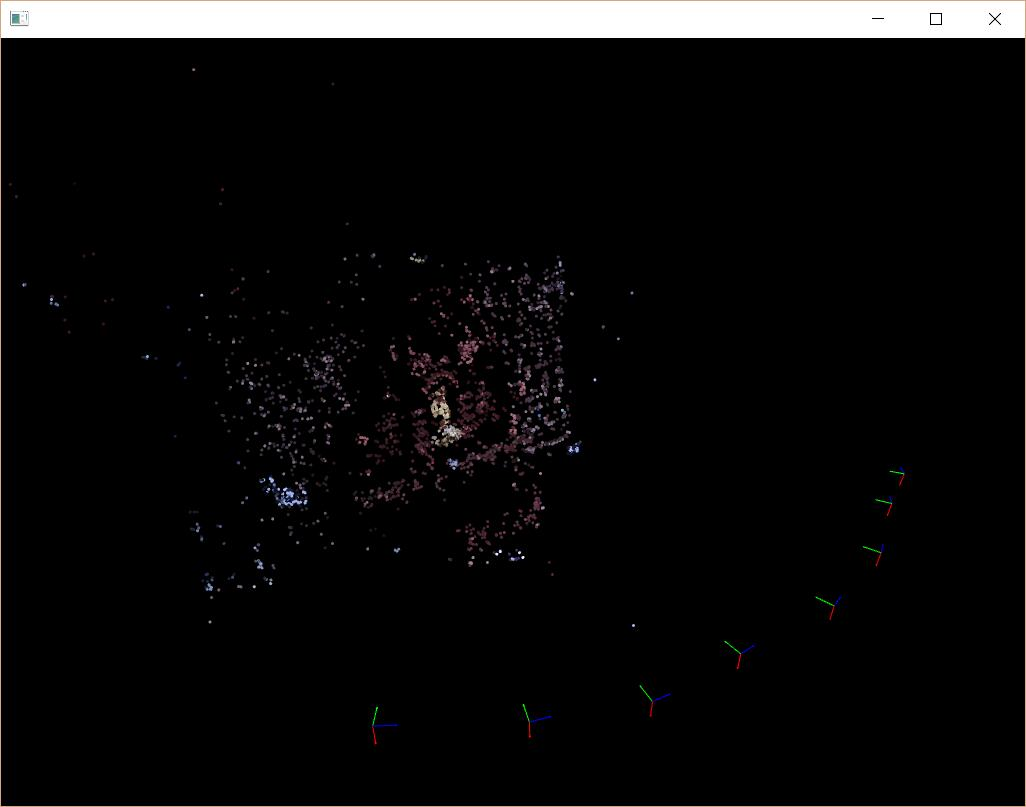## After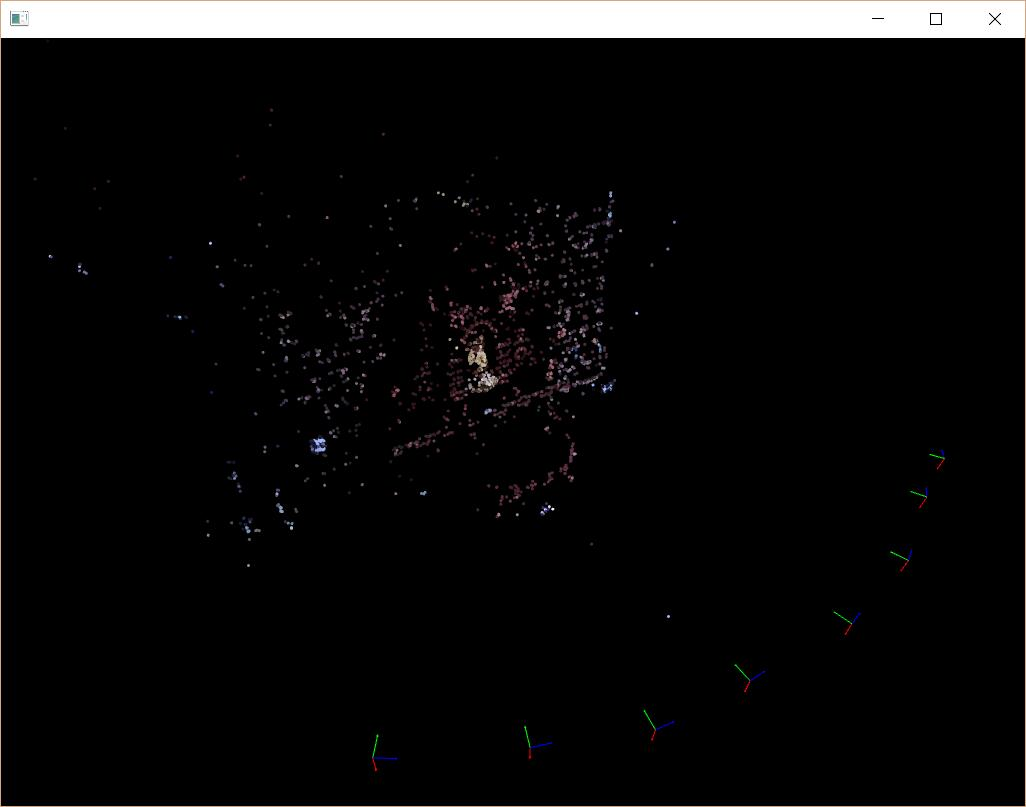## Before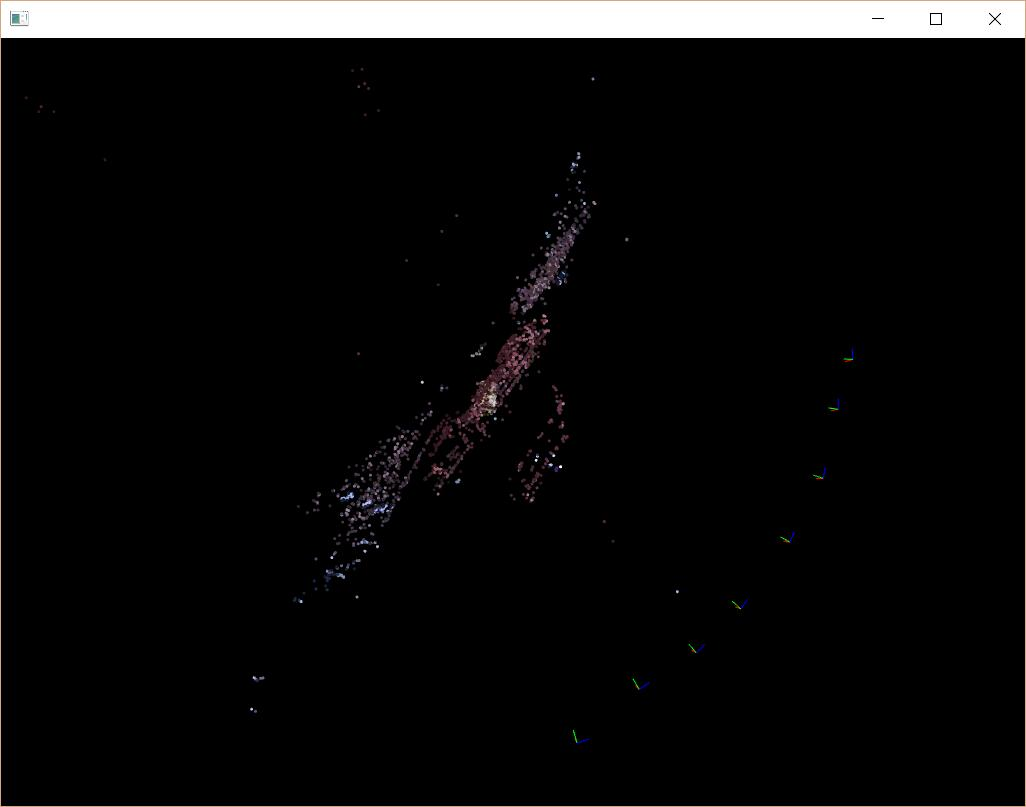## After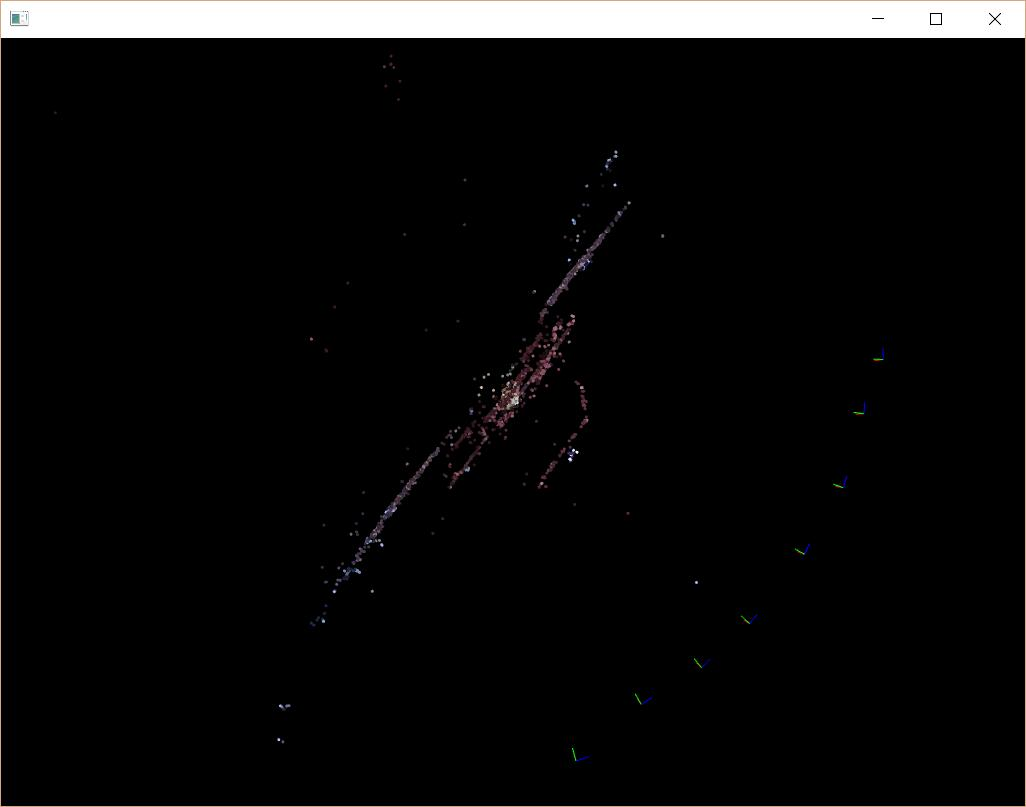## Statistics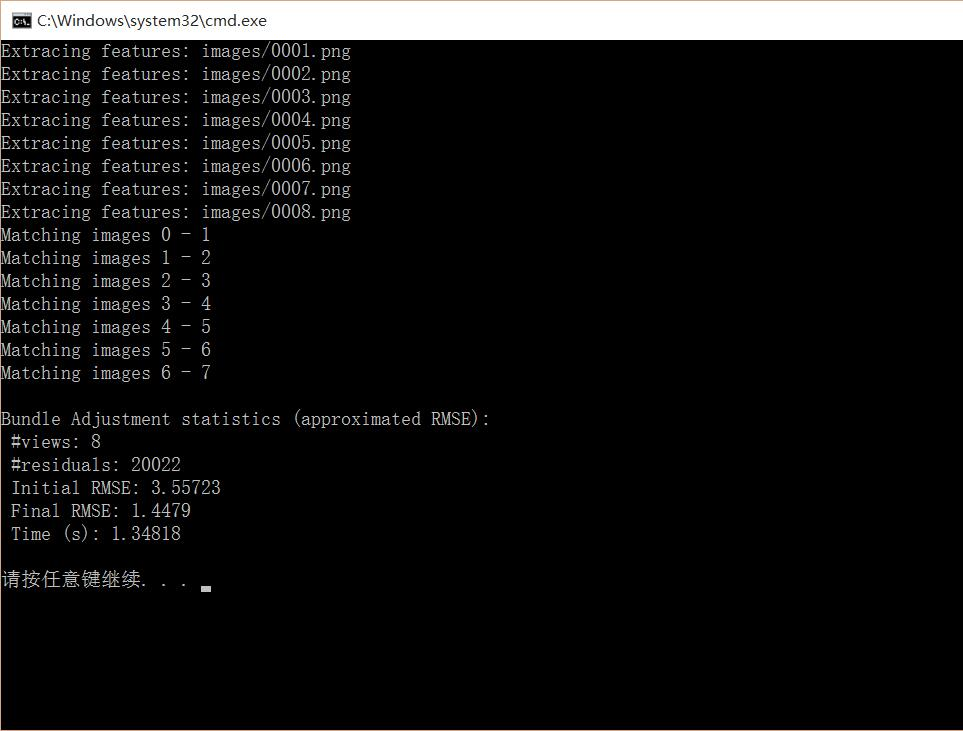# 结语

### MyBatis源码的学习（15）---sqlSession.selectList方法_itw_zhangzx02的博客-程序员资料_etsqlsession() .selectlist

sqlSession一共俩个实现类，我们这里分析默认的DefaultSqlSession类public &lt;E&gt; List&lt;E&gt; selectList(String statement, Object parameter, RowBounds rowBounds) { try { //ms对象代表我们的xml中的一条sql。例如：&lt;select...

### linux系统实验shell程序设计,实验三-shell脚本程序设计_李苦李的博客-程序员资料

《实验三-shell脚本程序设计》由会员分享，可在线阅读，更多相关《实验三-shell脚本程序设计(10页珍藏版)》请在人人文库网上搜索。1、实 验 报 告课程名称 Linux系统实践 实验项目 LINUX SHELL脚本程序设计 实验仪器 PC 系 别 计算机学院 专 业 网络工程 班级/学号 网1702/2017011463 学生姓名 孟启贤 实验日期 4.15 成 绩 指导教师 李艳平 实验...

### Best Practices for Speeding Up Your Web Site_longware_新浪博客_龙威的博客-程序员资料

Minimize HTTP RequestsUse a Content Delivery NetworkAdd an Expires or a Cache-Control HeaderGzip ComponentsPut Stylesheets at the TopPut Scripts at the BottomAvoid CSS Expre...

### 【安卓开发系列 -- APP】APP 性能优化 -- 启动优化_奋斗企鹅CopperSun的博客-程序员资料_应用启动性能优化

【安卓开发系列 -- APP】APP 性能优化 -- 启动优化【1】APP 启动优化的必要性原因 : 用户希望应用能够及时响应并快速加载，启动时间长的 APP 不能满足该期望；启动太慢的结果 : 体验效果差，用户流失，产品失败；【2】启动流程以及分类【2.1】开机启动流程注意，该流程图所示的启动过程为系统创建并启动应用的过程，一般不需要优化；【2.2】冷启动冷启动指应用从头开始启动，系统进程在冷启动后才创建应用进程，发生冷启动的情况包括应用自设备启动后或系统终止应用后.

### PostMessage()_丫丫afc的博客-程序员资料

PostMessage函数PostMessage是WindowsAPI(应用程序接口)中的一个常用函数，用于将一条消息放入到消息队列中。消息队列里的消息通过调用GetMessage和PeekMessage取得。函数功能该函数将一个消息放入（寄送）到与指定窗口创建的线程相联系消息队列里，不等待线程处理消息就返回，是异步消息模式。消息队列里的消息通过调用GetMessage和PeekMess...